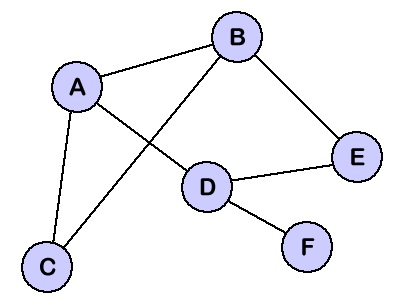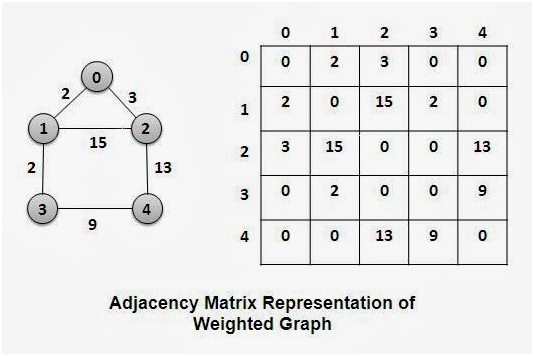# Insertion and deletion of nodes and edges in a graph using adjacency list

Submitted by Radib Kar, on July 07, 2020

A graph is a set of nodes or known number of vertices. When these vertices are paired together, we call it edges. An Edge is a line from one node to other. Every edge can have its cost or weight. Graphs are of two types Directed and Undirected. Directed graphs are the graphs in which the vertices are ordered and in undirected graphs the vertices are unordered.Image source: https://courses.cs.vt.edu/csonline/DataStructures/Lessons/Graphs/graph.gif

Node is a vertex in the graph at a position. The Line between two nodes is an edge. The Edge can have weight or cost associated with it.

Edge is the line connecting two nodes or a pair of nodes. An Adjacency matrix is a square matrix used to represent a finite graph. It contains the information about the edges and its cost. If the value at the Ith row and Jth column is zero, it means an edge do not exist between these two vertices. Else you got the edge and cost of that edge.C++ code:

```#include <bits/stdc++.h>
using namespace std;

{ //reference passed
//check if node alreday there
}
else {
unordered_set<int> st; //empty list
cout << "node added to the graph\n";
}
}

//to delete node
void delete_node(map<int, unordered_set<int> >& adj, int u)
{ //reference passed
//check if node is there or not
cout << "Node not present\n";
}
else {
//delete list of the node to be deleted
//delete from others node's list
if (it->second.find(u) != it->second.end()) {
it->second.erase(u);
}
}
cout << "node deleted from the graph\n";
}
}

{
//chcek if nodes are there or not
}
}
}
else {
cout << "Edge added to the graph\n";
}
else {
}
}
}

//to delete an edge
void delete_edge(map<int, unordered_set<int> >& adj, int u, int v)
{
//chcek if nodes are there or not
}
}
}
else {
cout << "Edge doesn't exist\n";
}
else {
cout << "Edge deleted from the graph\n";
}
}
}

//to print the graph by adjacency list
{
cout << "Empty graph\n";
return;
}

cout << "printing the graph in terms of adcacency list\n";
cout << "node     list of adjacency nodes\n";
for (auto & [ u, v ] : adj) {
cout << u << "      ";
if (v.size() == 0) {
cout << "Empty\n";
}
else {
for (auto& i : v) {
cout << i << " ";
}
cout << endl;
}
}
}

int main()
{
int option;
do {
cout << "1.press 1 to insert a node\n";
cout << "2.press 2 to delete a node\n";
cout << "3.press 3 to insert an edge\n";
cout << "4.press 4 to delete an edge\n";
cout << "5.print the graph via adjacency list\n";
cout << "6.exit\n";

cin >> option;
int node, u, v;
switch (option) {

cout << "input node to add(an integer denoting node no)\n";
cin >> node;
break;
case 2: //delete node

cout << "input node to delete(an integer denoting node no)\n";
cin >> node;
break;

cout << "input source and destination node to add an edge\n";
cout << "input source node\n";
cin >> u;
cout << "input destination node\n";
cin >> v;
break;
case 4: //delete edge

cout << "input source and destination node to delete an edge\n";
cout << "input source node\n";
cin >> u;
cout << "input destination node\n";
cin >> v;
break;
case 5: //print graph
break;
case 6: //exit
cout << "exiting...\n";
adj = {}; //deleting the graph, good practice to free memory
return 0;
break;
default:
cout << "Wrong input,only integers b/w 1-6:\n";
cout << "try again\n";
}
} while (true);

return 0;
}
```

Output:

```1.press 1 to insert a node
2.press 2 to delete a node
3.press 3 to insert an edge
4.press 4 to delete an edge
5.print the graph via adjacency list
6.exit
5
Empty graph
1.press 1 to insert a node
2.press 2 to delete a node
3.press 3 to insert an edge
4.press 4 to delete an edge
5.print the graph via adjacency list
6.exit
1
input node to add(an integer denoting node no)
4
1.press 1 to insert a node
2.press 2 to delete a node
3.press 3 to insert an edge
4.press 4 to delete an edge
5.print the graph via adjacency list
6.exit
3
input source and destination node to add an edge
input source node
4
input destination node
5
1.press 1 to insert a node
2.press 2 to delete a node
3.press 3 to insert an edge
4.press 4 to delete an edge
5.print the graph via adjacency list
6.exit
1
input node to add(an integer denoting node no)
5
1.press 1 to insert a node
2.press 2 to delete a node
3.press 3 to insert an edge
4.press 4 to delete an edge
5.print the graph via adjacency list
6.exit
3
input source and destination node to add an edge
input source node
4
input destination node
5
1.press 1 to insert a node
2.press 2 to delete a node
3.press 3 to insert an edge
4.press 4 to delete an edge
5.print the graph via adjacency list
6.exit
2
input node to delete(an integer denoting node no)
5
node deleted from the graph
1.press 1 to insert a node
2.press 2 to delete a node
3.press 3 to insert an edge
4.press 4 to delete an edge
5.print the graph via adjacency list
6.exit
5
printing the graph in terms of adcacency list
4      Empty
1.press 1 to insert a node
2.press 2 to delete a node
3.press 3 to insert an edge
4.press 4 to delete an edge
5.print the graph via adjacency list
6.exit
4
input source and destination node to delete an edge
input source node
4
input destination node
5
1.press 1 to insert a node
2.press 2 to delete a node
3.press 3 to insert an edge
4.press 4 to delete an edge
5.print the graph via adjacency list
6.exit
6
exiting...
```